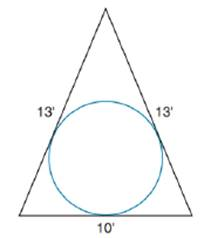Chapter 8.5, Problem 35EElementary Geometry For College St...

7th Edition
Alexander + 2 others
ISBN: 9781337614085

Solutions

Chapter
SectionElementary Geometry For College St...

7th Edition
Alexander + 2 others
ISBN: 9781337614085
Textbook Problem

A company logo on the side of a building shows an isosceles triangle with an inscribed circle. If the sides of triangle measure 10 ft, 13 ft and 13 ft, find the length of the radius of the inscribed circle.To determine

To find:

The length of radius of inscribed circle.

Explanation

Formula:

Area of a triangle with an inscribed circle:

If P is the perimeter of the triangle and r is the length of radius of its inscribed circle, then the area A of the triangle is given by,

A=12rP.

Area of triangle:

If a, b and c are lengths of sides of triangle, then area of triangle is given by the formula:

A=s(s-a)(s-b)(s-c)

Where s is the semi perimeter which is given by s=12(a+b+c).

Calculation:

To find the radius of inscribed circle, we will use the following formula:A=12rP, where A is the area of triangle, P is the perimeter of triangle and r is the radius of inscribed circle.

So, let’s find the area and perimeter of isosceles triangle.

The lengths of sides of triangle is given as 10 ft, 13 ft, 13 ft

Let a=10, b=13, c=13

Perimeter of triangle is sum of lengths of all sides of triangle.

Thus perimeter P=10+13+13=36 ft.

Let A be the area of triangle.

The semi perimeter is half of the perimeter of triangle. Thus, s=362=18 ft.

Let’s substitute these values in the formula to find area of triangle

Still sussing out bartleby?

Check out a sample textbook solution.

See a sample solution

The Solution to Your Study Problems

Bartleby provides explanations to thousands of textbook problems written by our experts, many with advanced degrees!

Get Started

Find more solutions based on key concepts